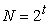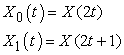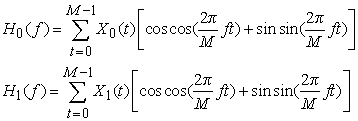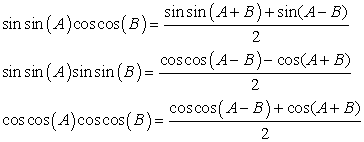﻿ Implementation of Parallel Fast Hartley Transform (FHT) Using Cuda

### Implementation of Parallel Fast Hartley Transform (FHT) Using Cuda

Hovhannes BantikyanOPEN ACCESSPEER-REVIEWED

## Implementation of Parallel Fast Hartley Transform (FHT) Using Cuda

Hovhannes BantikyanDepartment of Computer Systems and Informatics, State Engineering University of Armenia, Yerevan, Armenia

### Abstract

Implementation of Fast Hartley Transform in parallel manner on Graphics Processing Unit, using CUDA technology is presented in this paper. Calculating FHT in parallel, using multiple threads, gives us huge improvement in calculation speed. Developed CUDA based parallel algorithm, which experimental results compared with results of CPU based sequential algorithm. Edge detection algorithms can be speed up for large images, performing in frequency domain. Here experiments are done on various edge detection filters and different image sizes, using fast Hartlay transform.

### At a glance: Figures

12
Prev Next

• Bantikyan, Hovhannes. "Implementation of Parallel Fast Hartley Transform (FHT) Using Cuda." Journal of Computer Sciences and Applications 2.1 (2014): 6-8.
• Bantikyan, H. (2014). Implementation of Parallel Fast Hartley Transform (FHT) Using Cuda. Journal of Computer Sciences and Applications, 2(1), 6-8.
• Bantikyan, Hovhannes. "Implementation of Parallel Fast Hartley Transform (FHT) Using Cuda." Journal of Computer Sciences and Applications 2, no. 1 (2014): 6-8.

 Import into BibTeX Import into EndNote Import into RefMan Import into RefWorks

### 1. Introduction

Discrete transforms have a significant role in digital signal processing. They are used for many applications, such as image filtering, image reconstruction and image analysis. In this case Fourier Transform is the most widely used transformation. DFT converts the sampled function from its original domain (often time or position along a line) to the frequency domain. The input samples are complex numbers, and the output coefficients are complex as well.

A Discrete Hartley Transform (DHT) is a Fourier-related transform of discrete, periodic data similar to the Discrete Fourier Transform (DFT), with analogous applications in signal processing and related fields. Its main distinction from the DFT is that it transforms real inputs to real outputs, with no intrinsic involvement of complex numbers. In some applications it is more reasonable to use DHT when considering some of the advantages over DFT. So, DHT is a real-valued transform, it possesses the same formula for forward and inverse transform, it has a computational equivalence to DFT. Like FFT, Hartley Transform has a Fast algorithm too, which computes the DHT of lengthin.

The time needed for calculation can be reduced calculating FHT in parallel manner on GPU. GPU can process large volume data in parallel when working in single instruction multiple data (SIMD) mode. In November 2006, the Compute Unified Device Architecture (CUDA) which is specialized for compute intensive highly parallel computation is unveiled by NVIDIA.

Edge detection is an important task in image processing. It is a main tool in pattern recognition, image segmentation, and scene analysis. An edge detector is basically a high-pass filter that can be applied to extract the edge points in an image. In this paper, we will show classical time-domain edge detection filters and their frequency-domain analogues. We will show that using our GPU based FHT algorithm can gain in performance in edge detection process.

### 2. Fast Hartley Transform and Its Parallel Implementation with CUDA

The Hartley transform (HT) is defined like the Fourier transform with “cos+sin” instead of “cos+i•sin”. The (discrete) Hartley transform of x length-N sequence x is defined as(1)

The Hartley transform of a purely real sequence is purely real:(2)

The inverse transformation, which allows one to recover the X(t) from the H(f), is simply the DHT of H(f) multiplied by 1/N. Thus, the DHT is its own inverse, up to an overall scale factor.

In computing the DHT of a finite length signal with length N, gives us a time-complexity of O(N2). This means that for larger values of N, the computational time increases exponentially, which is not desirable. To make the DHT operation more practical, several FHT algorithms were proposed. Now let’s get down to business and see how the Hartley transform can be implemented efficiently as the FHT. The development is similar to that of the FFT. The heart of the algorithm is a computation whose data-flow diagram looks like a butterfly.

From now on we will be assuming that N is a power of two. Since N is even, the time sequence, X(t), which appears in the definition of the Hartley transform (Equation 1), can be divided into two intertwined sequences, X0 and X1, given by:That is, sequence X0 consists of the even-indexed values from sequence X and sequence X1 consists of the odd-indexed values. We will consider all indices to be interpreted modulo-N. But since X0 and X1 are defined in terms of 2t, they actually repeat modulo N/2. So consider X0 and X1 as sequences of length N/2 and let H0 and H1 be their Hartley transforms. Let M stand for N/2. Then, by the definition of the Hartley transform in Equation 1 we have:(3)

Using only the trig identities:(4)

we can verify that(5)

Keeping in mind that the Hartley transforms on the right side of Equation 5 repeat modulo N/2, we can recognize the following symmetry:(6)
Figure 1. The results of recursion Equations 5 and 6 are combined in this data-flow diagram. Blue represents terms with a cosine factor. Red represents terms with a sine factor. Black represents terms with no trig factor

Equations 5 and 6 taken together define the complete FHT butterfly computation, as diagrammed in Figure 10 .

One approach that we will discuss in this paper is Radix-2 FHT algorithm. In this case we assume that N is power of 2 (N=2v). The entire process is divided into log2N stages and in each stage N/2 two-point DHTs are performed. The computation involving each pair of data is called a butterfly. Radix-2 algorithm can be implemented as Decimation-in-time or Decimation-in-frequency algorithms. We will discuss Radix-2 DIT approach. As we want to implement algorithm without any recursions, we first need to compute bit-reversal permutation for N length signal. It will be done on host, that’s why for initial signal we will consider permuted signal, for example for N=8 length x{0, 1, 2, 3, 4, 5, 6, 7}, we will have x{0, 4, 2, 6, 1, 5, 3, 7}.

For Radix-2 FHT algorithm we have log2N stages for N length signal (for above example N=8 we have 3 stages). All butterflies in a stage can be performed in parallel and then at the end of the stage, the results can be gathered. Now all nodes can perform computation on the result of the first stage in parallel and output of the second stage can be gathered again and so on. Let us consider the decimation- in- time form of the length 8 Radix-2 algorithm. In the first stage, we have to perform eight separate 2-point DHTs. In the second stage, it visibly breaks into two separate 4-point DHTs and in the third stage, we have a single 8-point DHT. Figure 11 shows the parallel composition of this algorithm.

Texts in the ovals are the elements on which computation is performed and the numbers below the ovals are the threads that would perform the computation. Solid lines indicate communication. After every stage we call __syncthreads() of CUDA for the next Stage to be able to continue computing. In the decimation-in-frequency form, the arrows in the above figure need to be reversed and the stages should be numbered from bottom-up. So, this is just the inverse of decimation-in-time form. But the advantage of decimation-in-time form is that the result is already in the master node by the end of the last stage whereas in the case of decimation-in-frequency form, we need a result gathering phase after the last FHT stage.

### 3. Experimental Results

Experiments done on

1. CPU: Intel(R) Core(TM) i3-2100 3,10GHz.

2. GPU: GeForce GT 630, Max threads per block: 1024, Max blocks in kernel lunch: 65,535.

To do experiments and evaluate performance of GPU based FHT algorithm we choose one of FTH applications – edge detection. For edge detection process we took some well known filters. Here are edge detection filters we use for our experiments:

1. (average) Averaging filter.

2. (disk) Circular averaging filter (pillbox).

3. (gaussian) Gaussian lowpass filter.

4. (laplacian) Approximates the two-dimensional Laplacian operator.

5. (log) Laplacian of Gaussian filter.

6. (motion) Approximates the linear motion of a camera.

7. (prewitt) Prewitt horizontal edge-emphasizing filter.

8. (sobel) Sobel horizontal edge-emphasizing filter.

First we run filters in time domain, than in frequency – Hartley transformed domain. To filter images in frequency domain we do following steps:

1. calculate FHT of image.

2. pad filter matrix with zeros to fit image sizes.

3. calculate FHT of padded filter matrix.

4. multiply results of 1 and 3.

5. calculate inverse FHT of result 4.

To compare time domain and frequency domain filtering results, we run this procedure also on the image with noise. For that reason we add Gaussian white noise to the original image. So for experiments we will choose Lena image and the noisy version of it (Figure 12).

Figure 13 shows FHT based filtering results for above mentioned filters. First line is filtering results of original image, second line for image with noise.

In Table 1 are presented PSNRs of filtered images (original and noisy) for time domain and frequency domain.

#### Table 1. PSNRs of filtered images (original and noisy)Download asPowerPoint Slide

Veiw figureView current table in a new window

To evaluate performance we done experiments on images of different sizes (Figure 14). Here we will compare sequential FHT (on CPU) and parallel FHT (on GPU) algorithms. Figure 5 shows chart of edge detection sobel filter calculation time for classical filtering in time domain, using FHT on CPU and FHT on GPU.

Figure 5. Sobel filter (a) in time domain (b) using FHT CPU, (c) using FHT GPU

As we can see GPU based calculation give as huge gain in performance for large images.

### 4. Conclusion

As we can see, we gain in performance using parallel GPU algorithm. Parallelizing the sequential and simple FHT algorithms will be beneficial to control code complexity and minimize execution time of the process.

### Acknowledgement

I would like to thank Hakob Sarukhanyan for useful and pragmatic suggestions.

### References

  Bracewell R. N., The Hartley Transform, Oxford, Oxford Univ. Press, 1986, 168 pages.In article  Scott R., Doing Hartley smartly, EE Times-India, 2000.In article  Millane R. R., Analytic Properties of Hartley Transform and their Implications, IEEE, 1994.In article  Henning K., Maurico D., and Jurgen F., The Hartley transform in seismic imaging, GEOPHYSICS VOL. 66, NO. 4 (JULY-AUGUST 2001), Pages. 1251-1257.In article  Somasundaram M. Implementation and performance evaluation of parallel FFT algorithms.In article  B. Jähne., Digital Image Processing. 2005.In article  J. Sanders, E. Kandrot., CUDA by Example. 2010.In article  NVIDIA CUDA C Programming Guide. NVIDIA Corp. 2012.In article  H. Lensch, R. Strzodka., Massively Parallel Computing with Cuda. 2010.In article